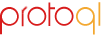A library designed for rapid assembly, querying, and interactive visual rendering of diagrams and common abstract mathematical structures.

Purpose

This library makes it possible to easily introduce specific kinds of interactive graph diagrams into HTML documents. It depends primarily on D3.js; it also supports initialization of jQuery-wrapped document elements as interactive diagrams.

Repository and Packages

The official repository is maintained on GitHub. The JavaScript package is also available on npm and the Python package is available on PyPI.

npm install protoql
pip install protoql

The latest version of the JavaScript library is also hosted at http://protoql.org/lib/protoql.js, although we recommend maintaining a copy on your own server at this time.

Example

Using the library is straightforward. Simply include a diagram description in document elements, and then apply the visualization constructor to those elements.

<script src="//d3js.org/d3.v3.min.js"></script>
<script src="//code.jquery.com/jquery-2.2.0.min.js"></script>
<script src="//protoql.org/lib/protoql.js"></script>
<div class="pql">
table([
[["dd`r``lorem`ipsum`dolor`sit amet"], ("s`r:lorem``consectetur"), ["dl:tempor``sed do`eiusmod tempor"]],
[null, ["dl``incididunt`ut labore"], null],
[("r:dolor``lorem`ipsum`dolor`sit amet"), ("r`uu``incididunt"), ["consectetur`adipiscing`elit"]]
])
</div>
</body>

The diagram above would render as shown below.
table([ [["dd`r``lorem`ipsum`dolor`sit amet"], ("s`r:lorem``consectetur"), ["dl:tempor``sed do`eiusmod tempor"]], [null, ["dl``incididunt`ut labore"], null], [("r:dolor``lorem`ipsum`dolor`sit amet"), ("r`uu``incididunt"), ["consectetur`adipiscing`elit"]] ])
The notation for tables being used above (inspired by the LaTeX xy package) is as follows:
• all nodes are strings describing the edges originating at that node and the node label, where:
• each individual arrow description string is of the form ddll (this is an example) and indicate the direction the arrow travel in the grid (e.g., d for "down"),
• the individual arrow strings are separated using a backquote character (i.e., `),
• the arrow descriptions and the label are separated using a double backquote (i.e., ``),
• the backquote character is also the newline separator for the label string, and
• circular nodes are delimited using (...), rectangular nodes are delimited using [...], and empty space is denoted using null;
• each row is represented using a list of node entries;
• the table is represented as a list of rows.
Supported diagram notations include table, relation, and graph. For example, the following will create a diagram with a small graph containing five nodes and three edges.

relation({'a','b','c'}, {'x','y'}, {('a','x'),('b','y'),('a','y')})

relation({'a','b','c'}, {'x','y'}, {('a','x'),('b','y'),('a','y')})
When using relation, it is possible to supply one set or two sets before supplying the edge set. In either case, two sets will be arranged side-by-side (two copies of the same set, or the two distinct sets, going from left to right), and the edges will be added between the two sets. When using graph, the nodes will be arranged from top to bottom, left to right as they are listed (roughly accommodating the dimensions of the diagram).

graph({'a','b','c','d','e'}, {('a','b'),('b','c'),('a','a'),('c','b')})

graph({'a','b','c','d','e'}, {('a','b'),('b','c'),('a','a'),('c','b')})
The set is optional in both relation and graph diagrams (if it is not supplied, it will be derived from the edge set). Adding the ! decorator fixes the diagram (zooming, panning, and dragging are disabled); this works for all three diagram types.

!graph({('a','b'),('b','c'),('a','a'),('c','b')})

!graph({('a','b'),('b','c'),('a','a'),('c','b')})
By using the # decorator, it is possible to create a diagram with a textbox allowing the user to edit the data from which the diagram is generated. Double-clicking any empty area in the diagram rebuilds the diagram using the data that currently appears in the textbox.

#graph({('a','b'),('b','c'),('a','a'),('c','b')})

#graph({('a','b'),('b','c'),('a','a'),('c','b')})